CAT  >  Test: CAT Quant 2019 ( Shift -1)

# Test: CAT Quant 2019 ( Shift -1)

Test Description

## 34 Questions MCQ Test Quantitative Aptitude (Quant) | Test: CAT Quant 2019 ( Shift -1)

Test: CAT Quant 2019 ( Shift -1) for CAT 2023 is part of Quantitative Aptitude (Quant) preparation. The Test: CAT Quant 2019 ( Shift -1) questions and answers have been prepared according to the CAT exam syllabus.The Test: CAT Quant 2019 ( Shift -1) MCQs are made for CAT 2023 Exam. Find important definitions, questions, notes, meanings, examples, exercises, MCQs and online tests for Test: CAT Quant 2019 ( Shift -1) below.
Solutions of Test: CAT Quant 2019 ( Shift -1) questions in English are available as part of our Quantitative Aptitude (Quant) for CAT & Test: CAT Quant 2019 ( Shift -1) solutions in Hindi for Quantitative Aptitude (Quant) course. Download more important topics, notes, lectures and mock test series for CAT Exam by signing up for free. Attempt Test: CAT Quant 2019 ( Shift -1) | 34 questions in 60 minutes | Mock test for CAT preparation | Free important questions MCQ to study Quantitative Aptitude (Quant) for CAT Exam | Download free PDF with solutions
 1 Crore+ students have signed up on EduRev. Have you?
Test: CAT Quant 2019 ( Shift -1) - Question 1

### Two cars travel the same distance starting at 10:00 am and 11:00 am, respectively, on the same day. They reach their common destination at the same point of time. If the first car travelled for at least 6 hours, then the highest possible value of the percentage by which the speed of the second car could exceed that of the first car is

Detailed Solution for Test: CAT Quant 2019 ( Shift -1) - Question 1 Let the speed of cars be a and b and the distance =d

Minimum time taken by 1st car = 6 hours,

For maximum difference in time taken by both of them, car 1 has to start at 10:00 AM and car 2 has to start at 11:00 AM.

Hence, car 2 will take 5 hours.

Hence a= d/6 and b = d/5

Hence the speed of car 2 will exceed the speed of car 1 by (d/5 – d/6)/d/6X 100

= (d/30 / d/6) x 100 = 20

Test: CAT Quant 2019 ( Shift -1) - Question 2

### If a1, a2 ... are in A.P., then,1/√a1 +√a2 + 1/√a2 + √a3 + …………… 1/√an +√an+1 is equal to

Detailed Solution for Test: CAT Quant 2019 ( Shift -1) - Question 2 We have, 1/√a1 +√a2 + 1/√a2 + √a3 + …………… 1/√an +√an+

Now, 1/ √a1 +√a2 = √a2 +√a1 / (√a2+√a1) (√a2+√a1)

(Multiplying numerator and denominator by (√a2 - √a1

= √a2 + √a3 / (a2 – a1)

= √a2 + √a1 /d

(where d is the common difference)

Similarly, 1/ √a2 + √a3 = (√a3 +√a2)/d and so on.

Then the expression 1/√a1 + √a2 +1/√a2+√a3 …….. + 1/√an + √an+1 can be written as

1/d (√a2 - √a1 + √a3 - √a3 + …………. √an+1 - √an)

=n/nd ((√an+1 - (√a1)

= n(√an+1 - √a1)/ an+1 - a1 [(an+1 - an = nd)]

= n/(√a1+ √an+1)

Test: CAT Quant 2019 ( Shift -1) - Question 3

### AB is a diameter of a circle of radius 5 cm. Let P and Q be two points on the circle so that the length of PB is 6 cm, and the length of AP is twice that of AQ. Then the length, in cm, of QB is nearest toOption~(a) 9.3(b) 7.8(c) 9.1(d) 8.5

Detailed Solution for Test: CAT Quant 2019 ( Shift -1) - Question 3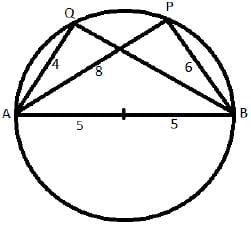Since AB is a diameter, AQB and APB’ will right angles.

In right triangle APB,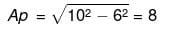Now, 2AQ = AP

=>AQ= 8/2=4

In right triangle AQB,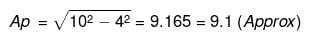Test: CAT Quant 2019 ( Shift -1) - Question 4

If (5.55) x = (0.555) y = 1000, then the value of 1/x – 1/y is

Detailed Solution for Test: CAT Quant 2019 ( Shift -1) - Question 4 We have, (5.55)x = (0.555)y = 1000

x(log10 555-2) = y(log10 555-3) = 3

Then, x(log10555-2) = 3…..(1)

y(log10555-3) = 3 ……(2)

From (1) and (2)

=>log10555= 3/x + 2 = 3/y +3

=> 1/x – 1/y = 1/3

Test: CAT Quant 2019 ( Shift -1) - Question 5

The income of Amala is 20% more than that of Bimala and 20% less than that of Kamala. If Kamala's income goes down by 4% and Bimala's goes up by 10%, then the percentage by which Kamala's income would exceed Bimala's is nearest to

Detailed Solution for Test: CAT Quant 2019 ( Shift -1) - Question 5 Assuming the income of Bimla = 100a, then the income of Amala will be 120a.

And the income of Kamala will be 120a*100/80=150a

If Kamala's income goes down by, 4%, then new income of Kamala = 150a-150a(4/100) = 150a-6a=144a

If Bimla's income goes up by 10 percent, her new income will be 100a+100a(10/100)=110a

=> Hence the Kamala income will exceed Bimla income by (144a-110a)*100/110a=31

Test: CAT Quant 2019 ( Shift -1) - Question 6

The wheels of bicycles A and B have radii 30 cm and 40 cm, respectively. While traveling a certain distance, each wheel of A required 5000 more revolutions than each wheel of B. If bicycle B traveled this distance in 45 minutes, then its speed, in km per hour, was

Detailed Solution for Test: CAT Quant 2019 ( Shift -1) - Question 6 Distance covered by A in 1 revolution = 2π*30 = 60π

Distance covered by B in 1 revolution = 2π*40 = 80π

Now, (5000+n)60π = 80πn

=> 15000= 4n-3n =>n=15000

Then distance travelled by B = 15000*80π cm = 12πkm

Hence, the speed = (12π X 60)/45= 16π

Test: CAT Quant 2019 ( Shift -1) - Question 7

The product of the distinct roots of | x2- x - 6 | = x + 2 is

Detailed Solution for Test: CAT Quant 2019 ( Shift -1) - Question 7 We have, |x2- x -6|= x + 2

=>|(x-3)(x+2)|=x+2

For x<-2, (3-x)(-x-2)=x+2

=> x-3=1 =>x = 4 (Rejected as x<-2)

For -2 ≤ x<3, (3-x)(x+2)=x+2

=>x=2,-2

For x≥ 3, (x-3)(x+2)=x+2 =>x=4

Hence the product =4*-2*2=-16

Test: CAT Quant 2019 ( Shift -1) - Question 8

In a race of three horses, the first beat the second by 11 metres and the third by 90 metres. If the second beat the third by 80 metres, what was the length, in metres, of the racecourse?

Detailed Solution for Test: CAT Quant 2019 ( Shift -1) - Question 8

Assuming the length of race course = x and the speed of three horses be a,b and c respectively.

Hence, x/a = (x-11)/b…. (1)

and x/a = (x – 90)/c …. (2)

Also, x/b = x – 80/c …. (3)

From 1 and 2, we get, (x-11)/b = (x-90)/c …. (4)

Dividing (3) by (4), we get, x – 11/x = x – 90/x-80

⇒ (x-11 )(x-80) = x(x-90)

⇒ 91x-90x=880
⇒ x=880

Test: CAT Quant 2019 ( Shift -1) - Question 9

If the population of a town is p in the beginning of any year then it becomes 3 + 2p in the beginning of the next year. If the population in the beginning of 2019 is 1000, then the population in the beginning of 2034 will be

Detailed Solution for Test: CAT Quant 2019 ( Shift -1) - Question 9 The population of town at the beginning of 1st year = p

The population of town at the beginning of 2nd year = 3+2p

The population of town at the beginning of 3rd year = 2(3+2p)+3 = 2*2p+2*3+3 =4p+3(1 +2)

The population of town at the beginning of 4th year = 2(2*2p+2*3+3)+3 = 8p+3(1+2+4)

Similarly population at the beginning of the nth year = 2n-1p+3(2n-1- 1) = 2n-1(p + 3)-3

The population in the beginning of 2019 is 1000, then the population in the beginning of 2034 will be (22034-2019) (1000 + 3)-3 = 215 (1003)-3

Test: CAT Quant 2019 ( Shift -1) - Question 10

Consider a function f satisfying f (x + y) = f (x) f (y) where x,y are positive integers, and f(1) = 2. If f(a + 1) +f (a + 2) +... + f(a + n) = 16 (2n -1) then a is equal to

Detailed Solution for Test: CAT Quant 2019 ( Shift -1) - Question 10

f(x + y)=f(x)f(y)

Hence, f(2) = f(1+1)=f(1)*f(1)=2*2=4

f(3)=f(2+1)=f(2)*f(1)=4*2=8

f(4)=f(3+1)=f(3)*f(1)=8*2=16

……… =>f(x)=2x

Now,f(a + 1)+f(a + 2) + ... + f(a + n) = 16(2n-1)

On putting n=1 in the equation we get, f(a+1)=16

⇒ f(a)*f(1)=16 (It is given that f (x + y) = f (x) f (y))

⇒ 2a*2=16

⇒ a=3

Test: CAT Quant 2019 ( Shift -1) - Question 11

Amala, Bina, and Gouri invest money in the ratio 3: 4: 5 in fixed deposits having respective annual interest rates in the ratio 6:5:4. What is their total interest income (in Rs) after a year, if Bina's interest income exceeds Amala's by Rs 250?

Detailed Solution for Test: CAT Quant 2019 ( Shift -1) - Question 11 Assuming the investment of Amala, Bina, and Gouri be 300x, 400x and 500x, hence the interest incomes will be 300x*6/100=18x, 400x*5/100=20x and 500x*4/100 = 20x

Given, Bina's interest income exceeds Amala by 20x-18x=2x=250 => x=125

Now, total interest income = 18x+20x+20x=58x = 58*125 = 7250

Test: CAT Quant 2019 ( Shift -1) - Question 12

For any positive integer n, let f (n) = n (n + 1) if n is even, and f (n) = n + 3 if n is odd. If m is a positive integer such that 8f (m + 1) - f (m) = 2, then m equals

Detailed Solution for Test: CAT Quant 2019 ( Shift -1) - Question 12

Assuming m is even, then 8f(m+1)-f(m)=2

m+1 will be odd

So, 8(m+1+3)-m(m+1)=2

=> 8m+32-m2– m=2

=> m2 − 7m − 30 = 0

=> m=10,-3

Rejecting the negative value, we get m=10

Assuming m is odd, m+1 will be even.

Then, 8(m+1) (m+2) – m – 3=2

=> 8(m2+3m+2) – m – 3=2

=> 8m2 + 23m +11=0

Discriminant for the equation = 232–4.8.11 = 177

Hence, the value of m will not be integral. Hence this case will be rejected.

Test: CAT Quant 2019 ( Shift -1) - Question 13

The product of two positive numbers is 616. If the ratio of the difference of their cubes to the cube of their difference is 157:3, then the sum of the two numbers is

Detailed Solution for Test: CAT Quant 2019 ( Shift -1) - Question 13 Assume the numbers are a and b, then ab=616

We have, a3 – b3 / (a - b)3 = 157/3

=> 3 (a3 − b3) = 157 (a3 − b3 + 3ab (b − a))

=> 154 (a3 — b3) + 3 * 157 * ab(b — a) = 0

=> 154 (a3 - b3) + 3 * 616 * 157 (b - a) = 0 (ab=616)

=>a3 - b3 + (3 x 4 x 157 (b - a)) (154*4=616)

=> (a - b) (a2 + b2 + ab) = 3 x 4 x 157 (a - b)

=>a2 + b2 + ab = 3x4x157

Adding ab=616 on both sides, we get

a2 + b2 + ab + ab = 3 x 4 x 157 + 616

=> (a + b)2 = 3 x 4 x 157 + 616 = 2500

=>a+b=50

Test: CAT Quant 2019 ( Shift -1) - Question 14

One can use three different transports which move at 10,20, and 30 kmph, respectively to reach from A to B. Amal took each mode of transport for 1/3 rd of his total journey time, while Bimal took each mode of transport for 1/3rd of the total distance. The percentage by which Bimal's travel time exceeds Amal's travel time is nearest to

Detailed Solution for Test: CAT Quant 2019 ( Shift -1) - Question 14 Assume the total distance between A and B as d and time taken by Amal = t

Since Amal travelled 1/3rd of his total journey time in different speeds

d = t/3 x 10 + t/3 x 20 + t/3X 30 = 20t

Total time taken by Bimal = d1/s1 + d2/s2 + d3/s3

= 20t/3 x 1/10 + 20t/3 x 1/20 + 20t/3 x 1/30 = 20t(6+3+2)/3x30 = 11/9t

Hence, the ratio of time taken by Bimal to time taken by Amal =11t/(9/t)= 9

Therefore, Bimal will exceed Amal's time by (11t/9 – t)/tx 100 = 22.22

Test: CAT Quant 2019 ( Shift -1) - Question 15

Meena scores 40% in an examination and after review, even though her score is increased by 50%, she fails by 35 marks. If her postreview score is increased by 20%, she will have 7 marks more than thepassing score. The percentage score needed for passing the examination is

Detailed Solution for Test: CAT Quant 2019 ( Shift -1) - Question 15 Assuming the maximum marks =100a, then Meena got 40a

After increasing her score by 50%, she will get 40a(1 +50/100)-60a

Passing score = 60a+35

Post review score after 20% increase = 60a*1.2=72a

=>Hence, 60a+35+7=72a

=>12a=42

=>a=3.5

=> maximum marks = 350 and passing marks = 210+35=245

=> Passing percentage = 245*100/350 = 70

Test: CAT Quant 2019 ( Shift -1) - Question 16

A person invested a total amount of Rs 15 lakh. A part of it was invested in a fixed deposit earning 6% annual interest, and the remaining amount was invested in two other deposits in the ratio 2:1, earning annual interest at the rates of 4% and 3%, respectively. If the total annual interest income is Rs 76000 then the amount (in Rs lakh) invested in the fixed deposit was

Detailed Solution for Test: CAT Quant 2019 ( Shift -1) - Question 16 Assuming the amount invested in the ratio,2:1 was 200x and 100x, then the fixed deposit investment = 1500000-300x

Hence, the interest = 200x*4/100 = 8x and 100x*3/100=3x

Interest from the fixed deposit = (1500000-300x)*6/100 = 90000-18x

Hence the total interest = 90000-18x+8x+3x=90000-7x =76000

=> 7x=14000

=>x=2000

Hence, the fixed deposit investment = 1500000 – 300*2000 = 900000 = 9 lakhs

Test: CAT Quant 2019 ( Shift -1) - Question 17

A club has 256 members of whom 144 can play football, 123 can play tennis, and 132 can play cricket. Moreover, 58 members can play both football and tennis, 25 can play both cricket and tennis, while 63 can play both football and cricket. If every member can play at least one game, then the number of members who can play only tennis is

Detailed Solution for Test: CAT Quant 2019 ( Shift -1) - Question 17 Assume the number of members who can play exactly 1 game = I

The number of members who can play exactly 1 game = II

The number of members who can play exactly 1 game = III

1+2II+3III = 144+123+132=399....(1)

I+II+III=256……(2)

=>11+2111=143…..(3)

Also, II+3III=58+25+63=146…..(4)

=> III = 3 (From 3 and 4)

=> II = 137

=> I = 116

The members who play only tennis = 123 – 58 – 25+3 = 43

Test: CAT Quant 2019 ( Shift -1) - Question 18

If a1 + a2 + a3 +.... + an = 3(2n+1 - 2), for every n ≥ 1, then a11 equals

Detailed Solution for Test: CAT Quant 2019 ( Shift -1) - Question 18 11th term of series = a11 = Sum of 11 terms - Sum of 10 terms = 3(211+1 - 2) - 3(210+1 - 2)

= 3(212 - 2 - 211 + 2) = 3(211)(2 - 1)= 3*211 = 6144

Positive Mark: 3

Negative Mark: 0

Test: CAT Quant 2019 ( Shift -1) - Question 19

The number of the real roots of the equation 2 cos(x(x + 1)) = 2x + 2-x is

Detailed Solution for Test: CAT Quant 2019 ( Shift -1) - Question 19 2 cos(x(x + 1)) = 2x + 2-x

The maximum value of LHS is 2 when cos(x(x + 1)) is 1 and the minimum value of RHS is 2 using AM ≥ GM

Hence LHS and RHS can only be equal when both sides are 2.

For LHS, cosx(x+1) =1 => x(x+1) =0 => x=0,-1

For RHS minimum value, x=0

Hence only one solution x=0

Test: CAT Quant 2019 ( Shift -1) - Question 20

At their usual efficiency levels, A and B together finish a task in 12 days. If A had worked half as efficiently as she usually does, and B had worked thrice as efficiently as he usually does, the task would have been completed in 9 days. How many days would A take to finish the task if she works alone at her usual efficiency?

Detailed Solution for Test: CAT Quant 2019 ( Shift -1) - Question 20 Assuming A completes a units of work in a day and B completes B units of work in a day and the total work = 1 unit

Hence, 12(a+b)=1……(1)

Also, 9(a/2+3b)=1

Using both equations, we get, 12(a+b)= 9(a/2+3b)

=> 4a+4b= 3a/2 +9b

5a/2 =5b

=> a=2b

Substituting the value of b in equation (1),

12(3a/2) = 1

=>a=1/18

Hence, the number of days required = 1/(1/18)=18

Test: CAT Quant 2019 ( Shift -1) - Question 21

In a class, 60% of the students are girls and the rest are boys. There are 30 more girls than boys. If 68% of the students, including 30 boys, pass an examination, the percentage of the girls who do not pass is

Detailed Solution for Test: CAT Quant 2019 ( Shift -1) - Question 21 Assuming the number of students=100x

Hence, the number of girls = 60x and the number of boys = 40x

We have, 60x-40x=30 =>x=1.5

The number of girls = 60*1.5=90

Number of girls that pass = 68x – 30=68*1.5-30 = 102 – 30=72

The number of girls who do not pass = 90 – 72=18

Hence the percentage of girls who do not pass = 1800/90-20

Test: CAT Quant 2019 ( Shift -1) - Question 22

In a circle of radius 11 cm, CD is a diameter and AB is a chord of length 20.5 cm. If AB and CD intersect at a point E inside the circle and CE has length 7 cm, then the difference of the lengths of BE and AE, in cm, is

Detailed Solution for Test: CAT Quant 2019 ( Shift -1) - Question 22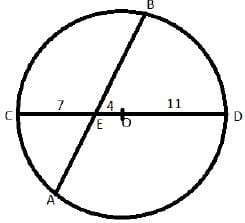B In figure AE*BE=CE*DE (The intersecting chords theorem)

=> 7*15=x(20.5-x)(Assuming AE=x)

=> 210=x(41 -2x)

=> 2x2-41x+210=0

=> x=10 or x=10.5

=> AE=10 or AE=10.5

Hence BE = 20.5-10=10.5 or BE = 20.5-10.5=10

Required difference= 10.5-10=0.5

Test: CAT Quant 2019 ( Shift -1) - Question 23

On selling a pen at 5% loss and a book at 15% gain, Karim gains Rs. 7. If he Sells the pen at 5% gain and the book at 10% gain, he gains Rs. 13. What is the cost price of the book in Rupees?

Detailed Solution for Test: CAT Quant 2019 ( Shift -1) - Question 23 Assuming the cost price of pen= 100p and the cost price of book = 100b

So, on selling a pen at 5% loss and a book at 15% gain, net gain = -5p+15b = 7 ....1

On selling the pen at 5% gain and the book,at 10% gain, net gain = 5p+10b = 13…2

Adding 1 and 2 we get, -25b=20

Hence 100b= 20*4=80,

Test: CAT Quant 2019 ( Shift -1) - Question 24

A chemist mixes two liquids 1 and 2. One litre of liquid 1 weighs 1 kg and one litre of liquid 2 weighs 800 gm. If half litre of the mixture weighs 480 gm, then the percentage of liquid 1 in the mixture, in terms of volume, is

Detailed Solution for Test: CAT Quant 2019 ( Shift -1) - Question 24 The weight/volume(g/L) for liquid 1 = 1000

The weight/volume(g/L) for liquid 2 = 800

The weight/volume(g/L) of the mixture = 480/(1/2) = 960

Using alligation the ratio of liquid 1 and liquid 2 in the mixture = (960-800)/(1000-960) = 160/40 = 4:1

Hence the percentage of liquid 1 in the mixture = 4*100/(4+1 )=80

Test: CAT Quant 2019 ( Shift -1) - Question 25

Ramesh and Gautam are among 22 students who write an examination. Ramesh scores 82.5. The average score of the 21 students other than Gautam is 62. The average score of all the 22 students is one more than the average score of the 21 students other than Ramesh. The score of Gautam is

Detailed Solution for Test: CAT Quant 2019 ( Shift -1) - Question 25 Assume the average of 21 students other than Ramesh = a

Sum of the scores of 21 students other than Ramesh = 21 a

Hence the average of 22 students = a+1

Sum of the scores of all 22 students = 22(a+1)

The score of Ramesh = Sum of scores of all 22 students - Sum of the scores of 21 students other than Ramesh = 22(a+1)-21 a=a+22 = 82.5 (Given)

=> a = 60.5

Hence, sum of the scores of all 22 students = 22(a+1) = 22*61.5 = 1353

Now the sum of the scores of students other than Gautam = 21*62 = 1302

Hence the score of Gautam = 1353 – 1302=51

Test: CAT Quant 2019 ( Shift -1) - Question 26

If m and n are integers such that (√2 )1934429m8n = 3n16m(√64 ) then m is

Detailed Solution for Test: CAT Quant 2019 ( Shift -1) - Question 26

We have, (√2 )1934429m8n = 3n16m(√64 )

Converting both sides in powers of 2 and 3, we get

219/2342332m23n = 3n24m26/4Comparing the power of 2 we get, 19/2 + 4 + 3n = 4m + 6/4

=> 4m=3n+12…. (1)

Comparing the power of 3 we get, 4 + 2m = n

Substituting the value of n in (1), we get

4m=3(4+2m)+12

=> m=-12

Test: CAT Quant 2019 ( Shift -1) - Question 27

Three men and eight machines can finish a job in half the time taken by three machines and eight men to finish the same job. If two machines can finish the job in 13 days, then how many men can finish the job in 13 days?

Detailed Solution for Test: CAT Quant 2019 ( Shift -1) - Question 27 Consider the work done by a man in a day = a and that by a machine = b

Since, three men and eight machines can finish a job in half the time taken by three machines and eight men to finish the same job, hence the efficiency will be double.

=> 3a+8b = 2(3b+8a)

=>13a=2b

Hence work done by 13 men in a day = work done by 2 machines in a day.

=> If two machines can finish the job in 13 days, then same work will be done 13 men in 13 days.

Hence the required number of men = 13

Test: CAT Quant 2019 ( Shift -1) - Question 28

Corners are cut off from an equilateral triangle T to produce a regular hexagon H. Then, the ratio of the area of H to the area of T is

Detailed Solution for Test: CAT Quant 2019 ( Shift -1) - Question 28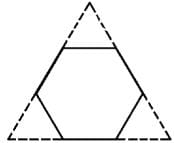The given figure can be divided into 9 regions or equilateral triangles of equal areas as shown below,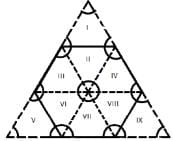Now the hexagon consists of 6 regions and the triangle consists of 9 regions.

Hence the ratio of areas = 6/9 =2:3

Test: CAT Quant 2019 ( Shift -1) - Question 29

Let x and y be positive real numbers such that

log5 (x + y) + log5 (x - y) = 3, and log2 y - log2 x = 1 - log2 3. Then xy equals

Detailed Solution for Test: CAT Quant 2019 ( Shift -1) - Question 29 We have, log5 (x + y) + log5 (x - y) = 3

=> x2 -y2 = 125…..1)

log2y - log2X = 1 - log23

=> y/x = 2/3

=> 2x=3y => x= 3y/2

On substituting the value of x in 1, we get

5x2/4 =125

=>y=10, x=15

Hence xy=150

Test: CAT Quant 2019 ( Shift -1) - Question 30

Let S be the set of all points (x, y) in the x-y plane such that |x|+|y|≤2 and |x| ≥ 1. Then, the area, in square units, of the regior represented by S equals

Detailed Solution for Test: CAT Quant 2019 ( Shift -1) - Question 30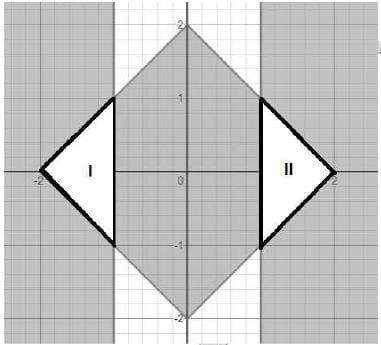Sum of the area of region I and II is the required area.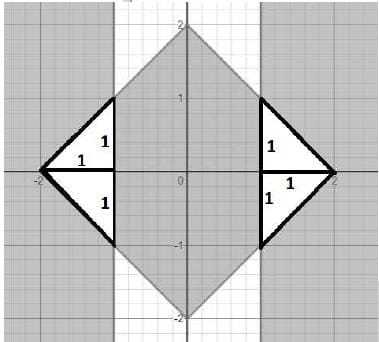Now, required area = 4x 1/2 x 1 x 1=2

Test: CAT Quant 2019 ( Shift -1) - Question 31

With rectangular axes of coordinates, the number of paths from (1,1) to (8,10) via (4, 6), where each step from any point (x, y) is either to (x, y+1) or to (x+1, y), is

Detailed Solution for Test: CAT Quant 2019 ( Shift -1) - Question 31 The number of paths from (1,1) to (8,10) via (4,6) = The number of paths from (1,1) to (4,6) * The number of paths from (4,6) to (8,10)

To calculate the number of paths from (1,1) to (4,6), 4-1 =3 steps in x-directions and 6-1 =5 steps in y direction

Hence the number of paths from (1,1) to (4,6) = (3+5)C3 – 56

To calculate the number of paths from (4,6) to (8,10), 8-4 =4 steps in x-directions and 10-6=4 steps in y direction

Hence the number of paths from (4,6) to (8,10) = (4+4)C4 = 70

The number of paths from (1,1) to (8,10) via (4,6) = 56*70=3920

Test: CAT Quant 2019 ( Shift -1) - Question 32

If the rectangular faces of a brick have their diagonals in the ratio 3 : 2√3 : √15, then the ratio of the length of the shortest edge of the brick to that of its longest edge is

Detailed Solution for Test: CAT Quant 2019 ( Shift -1) - Question 32 Assuming the dimensions of the brick are a, b and c and the diagonals are 3,2√3 and √15

Hence, a2 + b2 = 32… (1)

b2 + c2 = (2√3)2…. (2)

c2 + a2 = (√15)2….. (3)

Adding the three equations, 2(a2 + b2 + c2) = 9+12+15=36

=>a2 + b2 + c2 = 18 …… (4)

Subtracting (1) from (4), we get c2 = 9 =>c=3

Subtracting (2) from (4), we get a2 = 6 =>a=√6

Subtracting (3) from (4), we get b2 = 3 =>b=√3

The ratio of the length of the shortest edge of the brick to that of its longest edge is =√3/3 =1:√3

Test: CAT Quant 2019 ( Shift -1) - Question 33

The number of solutions to the equation | x | (6x2 + 1) = 5x2 is

Detailed Solution for Test: CAT Quant 2019 ( Shift -1) - Question 33

For x <0, -x(6x2 + 1) = 5x2

=> (6x2 + 1) = -5x

=> (6x2 + 5x + 1) = 0

=>(6x2 + 3x + 2x + 1) = 0

=> (3x+1)(2x+1)=0

=>x= -1/3 orx= -1/2

For x=0, LHS=RHS=0 (Hence, 1 solution)

For x >0, x(6x2 + 1) = 5x2

=> (6x2 - 5x + 1) = 0

=>(3x-1)(2x-1)=0

=> x = -1/3 or x= -1/2

Hence, the total number of solutions = 5

Test: CAT Quant 2019 ( Shift -1) - Question 34

Let T be the triangle formed by the straight line 3x + 5y - 45 = 0 and the coordinate axes. Let the circumcircle of T have radius of length L, measured in the same unit as the coordinate axes. Then, the integer closest to L is

Detailed Solution for Test: CAT Quant 2019 ( Shift -1) - Question 34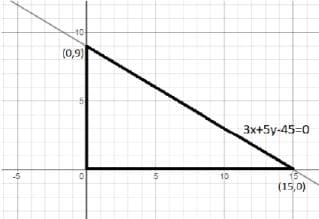In any right triangle, the circumradius is half of the hypotenuse.

Here, L= 1/2 * the length of the hypotenuse = ½ (√152 +92= ½ *√306

= ½ x 17.49 = 8.74

Hence, the integer close to L = 9

## Quantitative Aptitude (Quant)

163 videos|163 docs|131 tests
 Use Code STAYHOME200 and get INR 200 additional OFF Use Coupon Code
Information about Test: CAT Quant 2019 ( Shift -1) Page
In this test you can find the Exam questions for Test: CAT Quant 2019 ( Shift -1) solved & explained in the simplest way possible. Besides giving Questions and answers for Test: CAT Quant 2019 ( Shift -1), EduRev gives you an ample number of Online tests for practice

## Quantitative Aptitude (Quant)

163 videos|163 docs|131 tests

### How to Prepare for CAT

Read our guide to prepare for CAT which is created by Toppers & the best Teachers